Question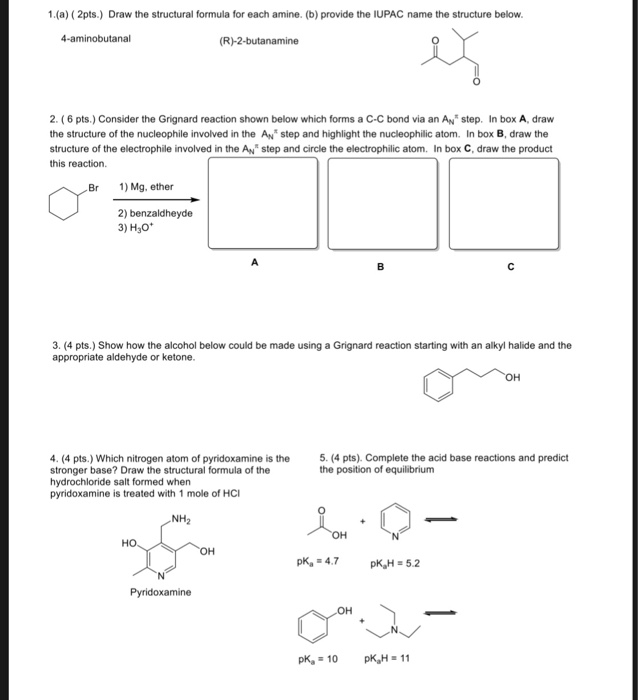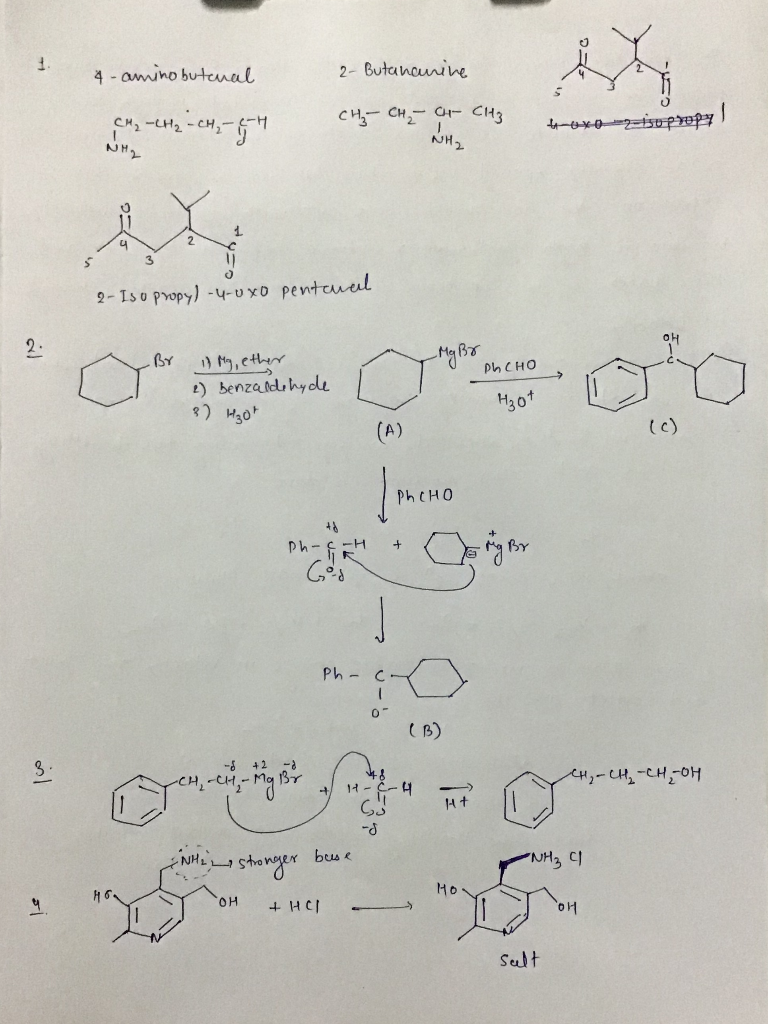#### Earn Coins

Coins can be redeemed for fabulous gifts.

Similar Homework Help Questions
• ### Draw a structural formula for the major organic product of the following reaction: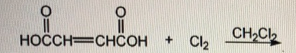Draw a structural formula for the major organic product of the following reaction:Electrophilic addition of HBr to alkenes yields a bromoalkane. The reaction begins with an attack on the hydrogen of the electrophilic HBr by the electrons of the double bond to give a carbocation. This step follows Markovnikov's rule with the electrophilic H atom adding to the sp2 carbon containing the most hydrogens, leading to the formation of the most stable carbocation (1° < 2° < 3°). If possible, a...

• ### Draw a structural formula for the major organic product of the reaction shown.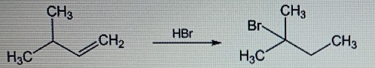Electrophilic addition of HBr to alkenes yields a bromoalkane. The reaction begins with an attack on the hydrogen of the electrophilic HBr by the electrons of the double bond to give a carbocation. This step follows Markovnikov's rule with the electrophilic H atom adding to the sp2 carbon containing the most hydrogens, leading to the formation of the most stable carbocation (1° < 2° < 3°). If possible, a 1,2-shift of either a neighboring hydride or methyl group can occur...

• ### 1) Draw the full structural formula, the condensed structural formula, and the line structure of each...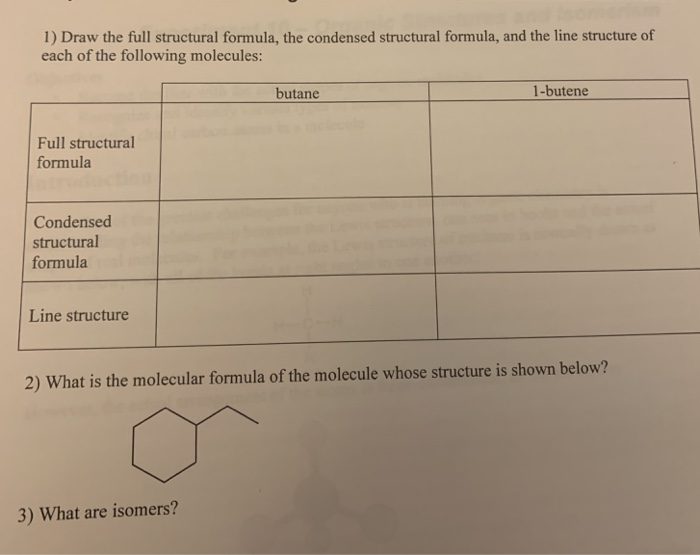1) Draw the full structural formula, the condensed structural formula, and the line structure of each of the following molecules: butane 1-butene Full structural formula Condensed structural formula Line structure 2) What is the molecular formula of the molecule whose structure is shown below? 3) What are isomers?

• ### Please include complete answers only, thank you. a. Draw the structural formula of each of the...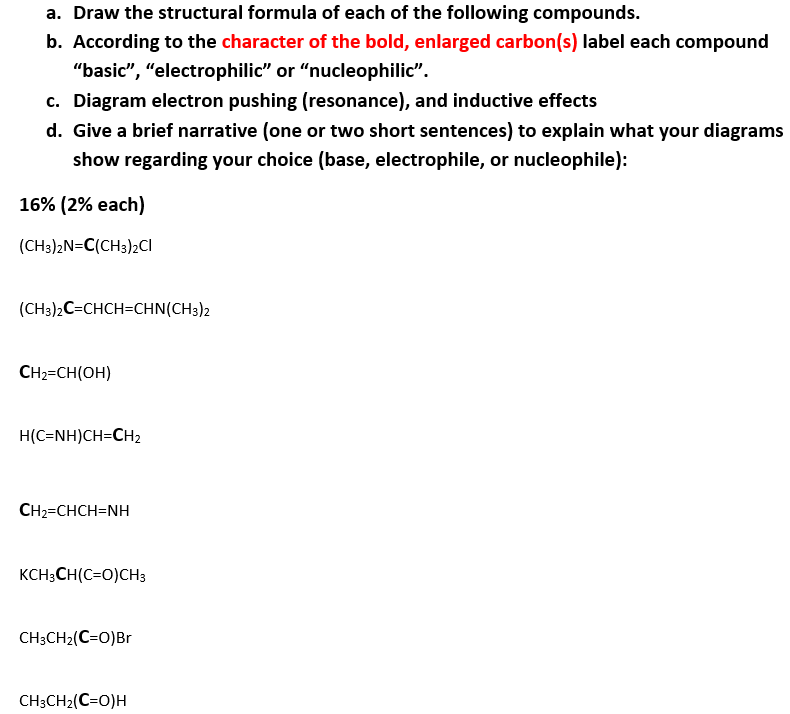Please include complete answers only, thank you. a. Draw the structural formula of each of the following compounds. b. According to the character of the bold, enlarged carbon(s) label each compound "basic", "electrophilic" or "nucleophilic". c. Diagram electron pushing (resonance), and inductive effects d. Give a brief narrative (one or two short sentences) to explain what your diagrams show regarding your choice (base, electrophile, or nucleophile): 16% (2% each) (CH3)2N=C(CH3)2CI (CH3)2C=CHCH=CHN(CH3)2 CH2=CH(OH) H(C=NH)CH=CH2 CH2=CHCHENH KCH3CH(C=O)CH3 CH3CH2(C=O)Br CH3CH2(C=O)H

• ### a. Draw the structural formula of each of the following compounds. b. According to the character...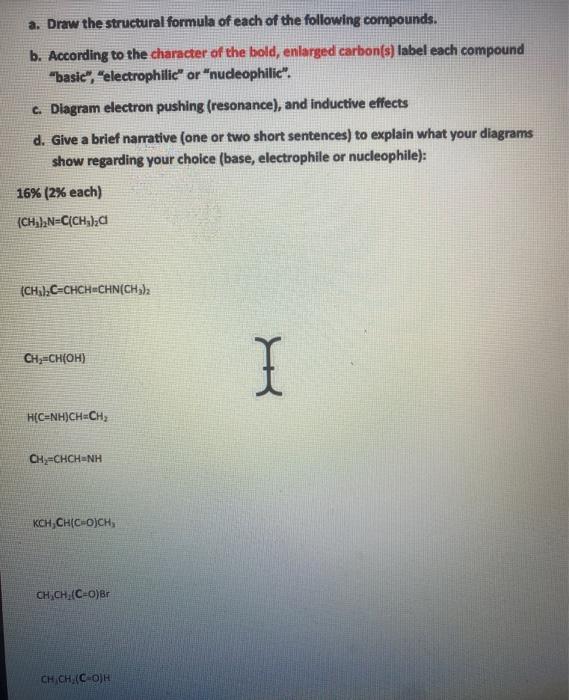a. Draw the structural formula of each of the following compounds. b. According to the character of the bold, enlarged carbon(s) label each compound "basic", "electrophilic" or "nudeophilic". c. Diagram electron pushing (resonance), and inductive effects d. Give a brief narrative (one or two short sentences) to explain what your diagrams show regarding your choice (base, electrophile or nucleophile): 16% (2% each) (CH)2N=C(CH4)2C (CH, C=CHCH=CHN(CH 3 CHCH(OH) I HỌC=NH)CH=CH2 CH2=CHCHENH KCH,CH(CO)CH CH CH(C=O)Br CH CH(CO)H

• ### draw a structural formula for the alkene you would use to prepare the alchol shown by...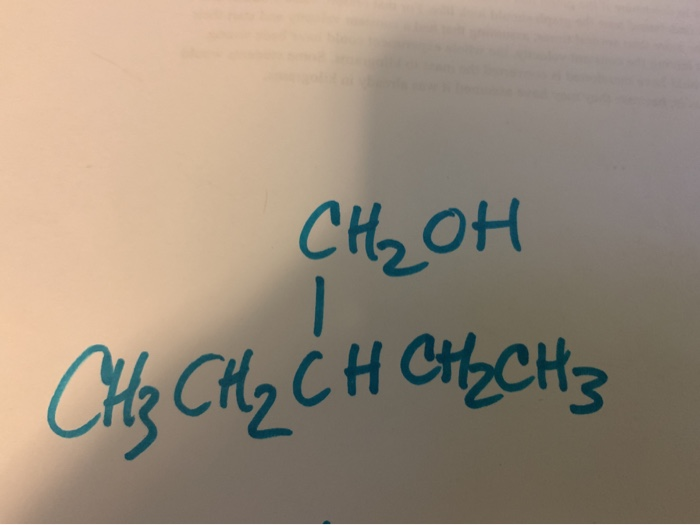draw a structural formula for the alkene you would use to prepare the alchol shown by hydroboration/oxidation. draw the structure of the makor organic product of the reaction below. when a new chirality center is created, isomers can result. For the reaction below, draw all of the major products using hashed and wedged bonds to show stereochemistry. please answer all three questions and box the answer. се, он Се си, ён снен; KOH + CHCh C + che en mer

• ### 2-methylocta-4,6-dien-1-amine Assignment 1: Draw your molecule from IUPAC name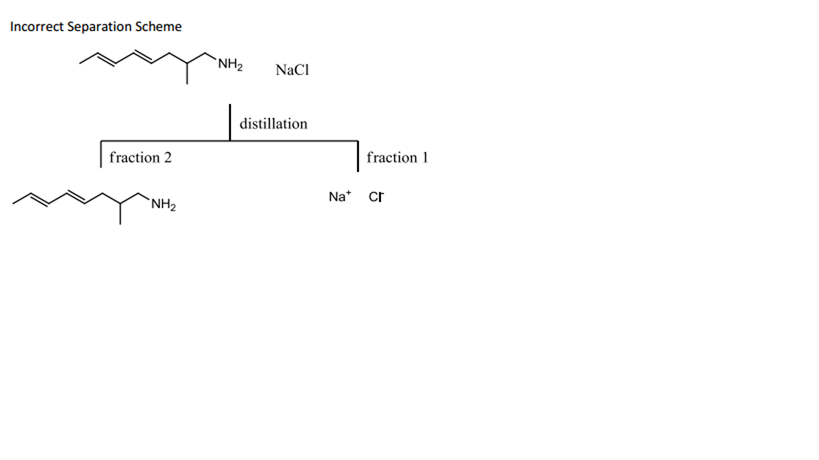2-methylocta-4,6-dien-1-amineAssignment 1: Draw your molecule from IUPAC name Using the given IUPAC name, draw your molecule Complete the cover page (p.1) of your portfolio with the following:o Structural formula of the moleculeo IUPAC name Use a thin, clear binder or report cover to protect the portfolio Submit the following in the binder:o Cover page as described above (page 1)Assignment 2: Formula, Molar Mass and Functional Groups On a page titled Expanded Structural Formula draw the expanded structure of themolecule.o Show...

• ### 2. Draw the structural formula for a- D-glucosyl-(1->6)-D-mannosamine, and circle the part of this structure that...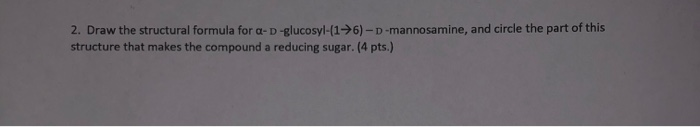2. Draw the structural formula for a- D-glucosyl-(1->6)-D-mannosamine, and circle the part of this structure that makes the compound a reducing sugar. (4 pts.)

• ### 3. Acids and Bases. (15 pts) (a) Circle the weakest acid in each series shown below (9 pts). Seri...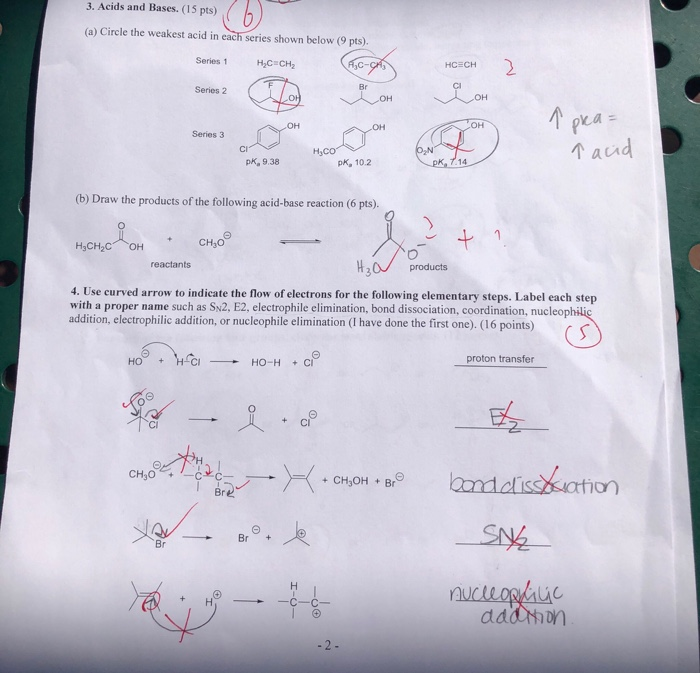poease answer and explain all. thank you! 3. Acids and Bases. (15 pts) (a) Circle the weakest acid in each series shown below (9 pts). Series 1 HC CH2 Series 2 OH он OH Series 3 Cl ai pk, 9.38 pk, 10.2 (b) Draw the products of the following acid-base reaction (6 pts). CH3O reactants products 4. Use curved arrow to indieate the flow of electrons for the following elementary steps. Label each step with a proper name such as...

• ### 1. The synthesis of Grignard reagent is challenging. a) Draw the product that is expected to...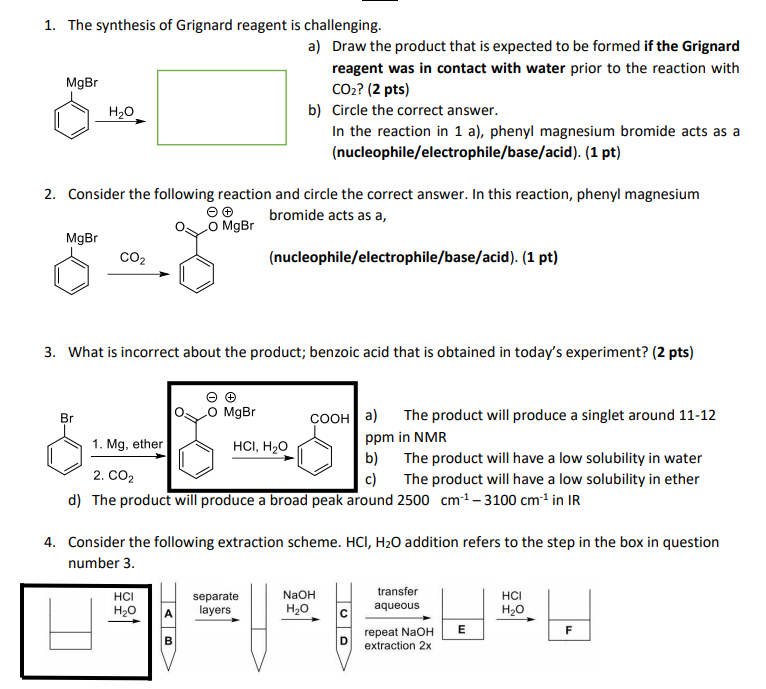1. The synthesis of Grignard reagent is challenging. a) Draw the product that is expected to be formed if the Grignard reagent was in contact with water prior to the reaction with MgBr CO2? (2 pts) b) Circle the correct answer. In the reaction in 1 a), phenyl magnesium bromide acts as a (nucleophile/electrophile/base/acid). (1 pt) Consider the following reaction and circle the correct answer. In this reaction, phenyl magnesium bromide acts as a, Oo MgBr MgBr (nucleophile/electrophile/base/acid). (1 pt)...